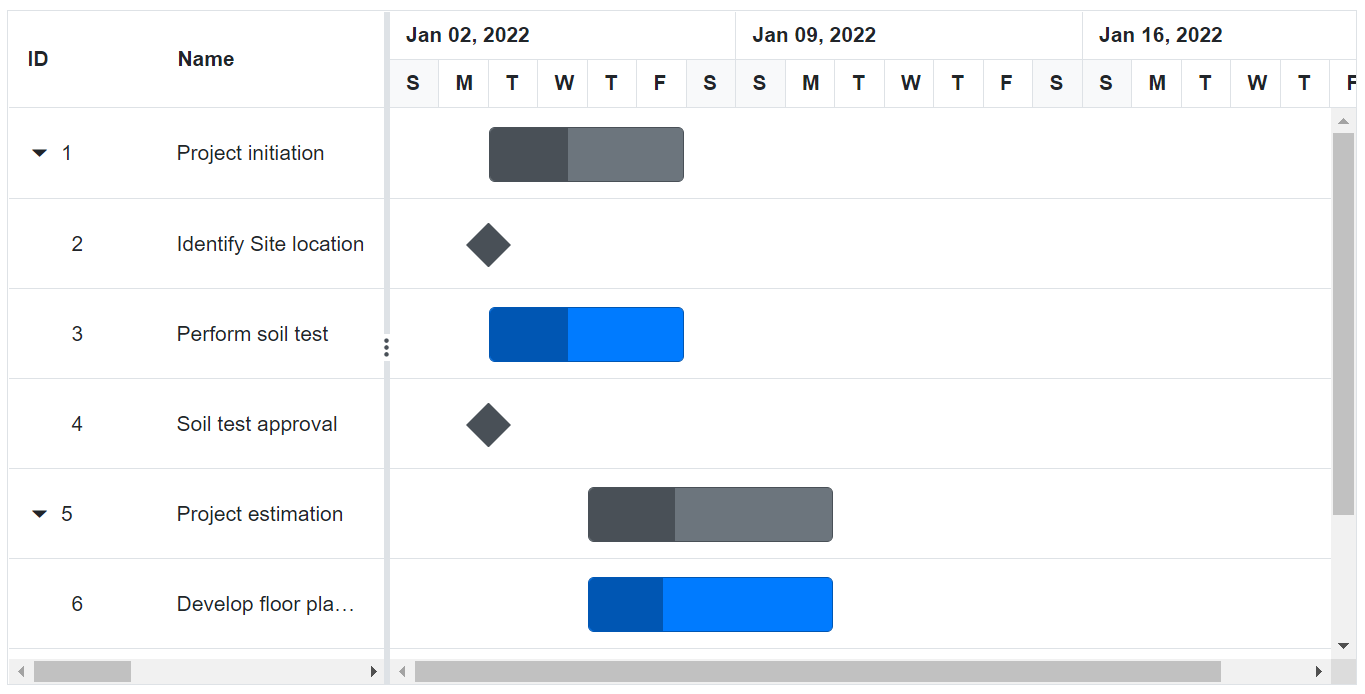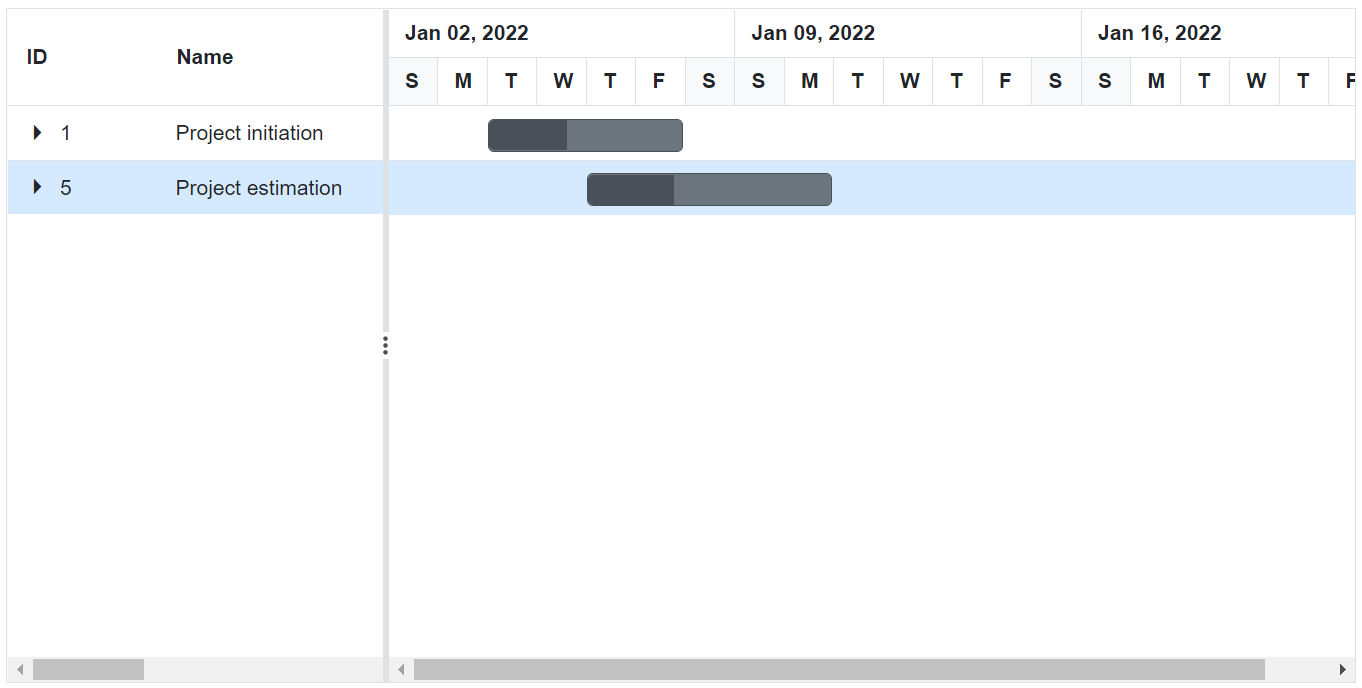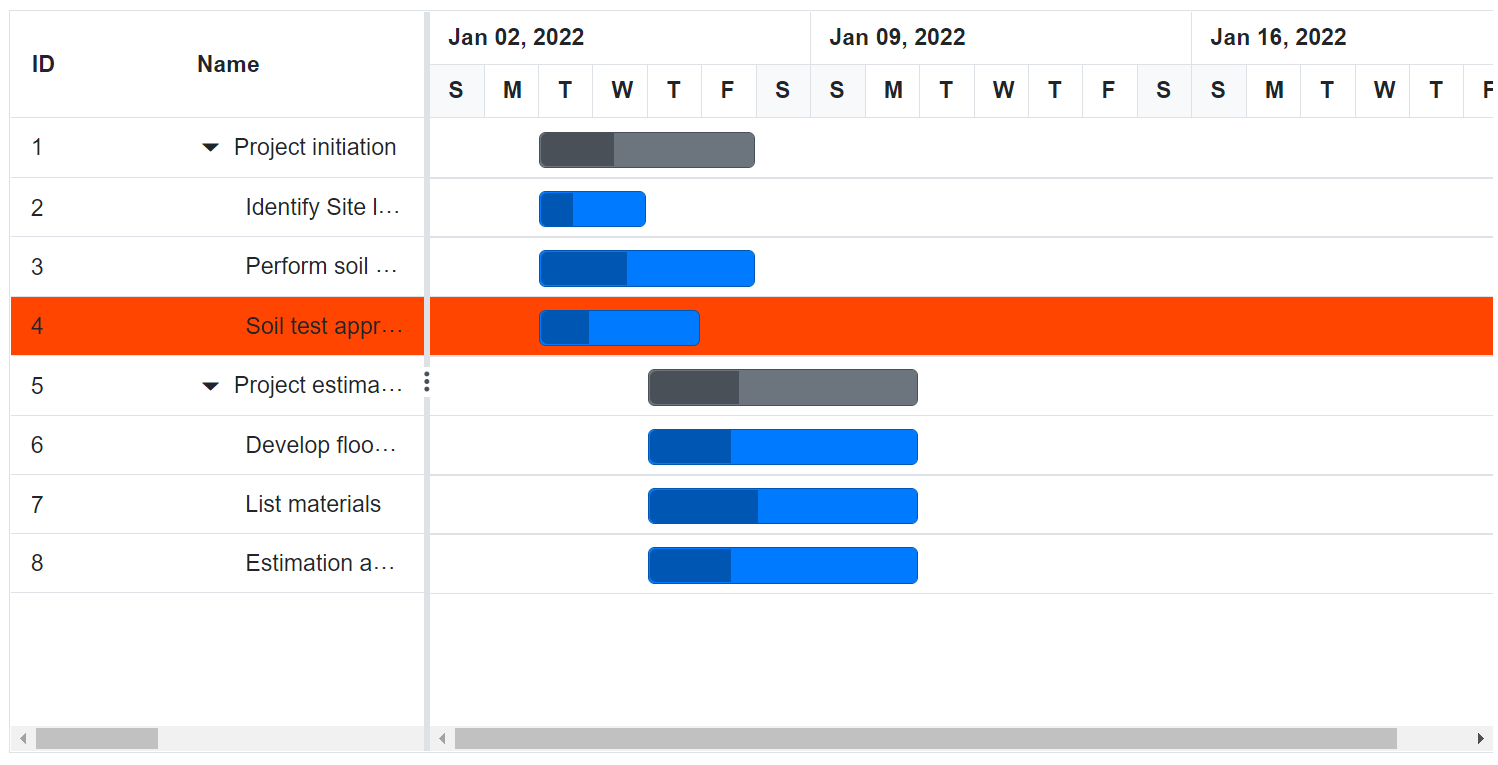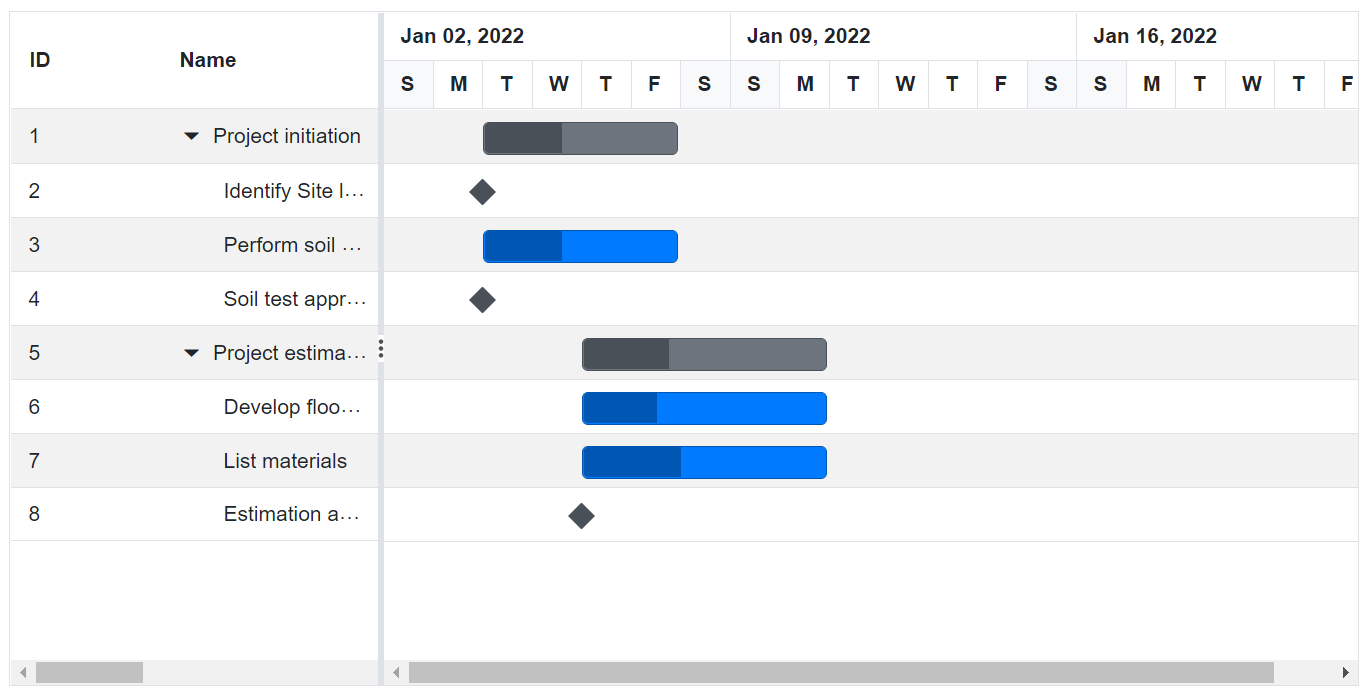Rows in Blazor Gantt Chart Component

17 Sep 202124 minutes to read

Row represents a task information from the data source, and it is possible to perform the following actions in Gantt Chart rows.

Row Height

It is possible to change the height of the row in Gantt Chart by setting row height in pixels to the RowHeight property. The following code example explains how to change the row height in Gantt Chart at load time.

@using Syncfusion.Blazor.Gantt
</SfGantt>

@code{
protected override void OnInitialized()
{
}

{
public int TaskId { get; set; }
public string TaskName { get; set; }
public DateTime StartDate { get; set; }
public DateTime EndDate { get; set; }
public string Duration { get; set; }
public int Progress { get; set; }
}

StartDate = new DateTime(2019, 04, 02),
EndDate = new DateTime(2019, 04, 21),
StartDate = new DateTime(2019, 04, 02),
Duration = "0",
Progress = 30,
},
StartDate = new DateTime(2019, 04, 02),
Duration = "4",
Progress = 40,
},
StartDate = new DateTime(2019, 04, 02),
Duration = "0",
Progress = 30
},
})
},
StartDate = new DateTime(2019, 04, 02),
EndDate = new DateTime(2019, 04, 21),
TaskName = "Develop floor plan for estimation",
StartDate = new DateTime(2019, 04, 04),
Duration = "3",
Progress = 30,
},
StartDate = new DateTime(2019, 04, 04),
Duration = "3",
Progress = 40
},
StartDate = new DateTime(2019, 04, 04),
Duration = "0",
Progress = 30,
}
})
}
};

}
}Expand or Collapse Row

In Gantt Chart parent tasks are expanded/collapsed by using expand/collapse icons, expand all/collapse all toolbar items and by using public methods. By default all tasks in Gantt Chart was rendered in expanded state but we can change this status through properties below.

All tasks available in Gantt Chart was rendered in collapsed state by setting CollapseAllParentTasks property as true. The following code example shows how to use this property.

@using Syncfusion.Blazor.Gantt
Duration="Duration" Progress="Progress" ParentID="ParentId">
</SfGantt>

@code{
protected override void OnInitialized()
{
}

{
public int TaskId { get; set; }
public string TaskName { get; set; }
public DateTime StartDate { get; set; }
public DateTime EndDate { get; set; }
public string Duration { get; set; }
public int Progress { get; set; }
public int? ParentId { get; set; }
}

StartDate = new DateTime(2019, 04, 02),
EndDate = new DateTime(2019, 04, 21)
},
StartDate = new DateTime(2019, 04, 02),
Duration = "0",
Progress = 30,
ParentId = 1
},
StartDate = new DateTime(2019, 04, 02),
Duration = "4",
Progress = 40,
ParentId = 1
},
StartDate = new DateTime(2019, 04, 02),
Duration = "0",
Progress = 30,
ParentId = 1
},
StartDate = new DateTime(2019, 04, 02),
EndDate = new DateTime(2019, 04, 21)
},
TaskName = "Develop floor plan for estimation",
StartDate = new DateTime(2019, 04, 04),
Duration = "3",
Progress = 30,
ParentId = 5
},
StartDate = new DateTime(2019, 04, 04),
Duration = "3",
Progress = 40,
ParentId = 5
},
StartDate = new DateTime(2019, 04, 04),
Duration = "0",
Progress = 30,
ParentId = 5
}
};
}
}Customize Expand and Collapse action

On expand action Expanding and Expanded event will be triggered with current expanding row’s information. Similarly on collapse action Collapsing and Collapsed event will be triggered. Using this events and it’s arguments we can customize the expand/collapse action. The following code example shows how to prevent the particular row from expand/collapse action using Expanding and Collapsing event.

@using Syncfusion.Blazor.Gantt
</SfGantt>

@code{
{
args.Cancel = true;
}
}
{
args.Cancel = true;
}
}
protected override void OnInitialized()
{
}

{
public int TaskId { get; set; }
public string TaskName { get; set; }
public DateTime StartDate { get; set; }
public DateTime EndDate { get; set; }
public string Duration { get; set; }
public int Progress { get; set; }
}

{
StartDate = new DateTime(2019, 04, 02),
EndDate = new DateTime(2019, 04, 21),
StartDate = new DateTime(2019, 04, 02),
Duration = "0",
Progress = 30,
},
StartDate = new DateTime(2019, 04, 02),
Duration = "4",
Progress = 40,
},
StartDate = new DateTime(2019, 04, 02),
Duration = "0",
Progress = 30
},
})
},
StartDate = new DateTime(2019, 04, 02),
EndDate = new DateTime(2019, 04, 21),
TaskName = "Develop floor plan for estimation",
StartDate = new DateTime(2019, 04, 04),
Duration = "3",
Progress = 30,
},
StartDate = new DateTime(2019, 04, 04),
Duration = "3",
Progress = 40
},
StartDate = new DateTime(2019, 04, 04),
Duration = "0",
Progress = 30,
}
})
}
};

}
}

Drag and Drop

You can dynamically rearrange the rows in the Gantt Chart component by using the AllowRowDragAndDrop property. Using this property, row drag and drop can be enabled or disabled in Gantt. Using this feature, rows can be dropped at above and below as a sibling or child to the existing rows

@using Syncfusion.Blazor.Gantt
<SfGantt DataSource="@TaskCollection" Height="450px" Width="900px" TreeColumnIndex="1" AllowRowDragAndDrop="true">
ParentID="ParentId">
</SfGantt>

@code{
protected override void OnInitialized()
{
}
{
public int TaskId { get; set; }
public string TaskName { get; set; }
public DateTime StartDate { get; set; }
public DateTime EndDate { get; set; }
public string Duration { get; set; }
public int Progress { get; set; }
public int? ParentId { get; set; }
}
StartDate = new DateTime(2019, 03, 28),
EndDate = new DateTime(2019, 07, 28),
Duration="4"
},
StartDate = new DateTime(2019, 03, 29),
Progress = 30,
ParentId = 1,
Duration="2",
},
StartDate = new DateTime(2019, 03, 29),
ParentId = 1,
Duration="4"
},
StartDate = new DateTime(2019, 03, 29),
Duration = "4",
Progress = 30,
ParentId = 1
},
StartDate = new DateTime(2019, 03, 29),
EndDate = new DateTime(2019, 04, 2),
Duration="4",
},
TaskName = "Develop floor plan for estimation",
StartDate = new DateTime(2019, 03, 29),
Duration = "3",
Progress = 30,
ParentId = 5
},
StartDate = new DateTime(2019, 04, 01),
Duration = "3",
Progress = 30,
ParentId = 5
},
StartDate = new DateTime(2019, 04, 01),
Duration = "2",
ParentId = 5
}
};
}
}

Multiple Row Drag and Drop

Gantt also supports dragging multiple rows at a time and drop them on any rows above, below, or at child positions. In Gantt, you can enable the multiple drag and drop by setting the GanttSelectionSettings.Type to Multiple and you should enable the AllowRowDragAndDrop property.

@using Syncfusion.Blazor.Gantt
<SfGantt DataSource="@TaskCollection" Height="450px" Width="900px" TreeColumnIndex="1" AllowRowDragAndDrop="true">
ParentID="ParentId">
<GanttSelectionSettings Type="Syncfusion.Blazor.Grids.SelectionType.Multiple"></GanttSelectionSettings>
</SfGantt>

@code{
protected override void OnInitialized()
{
}
{
public int TaskId { get; set; }
public string TaskName { get; set; }
public DateTime StartDate { get; set; }
public DateTime EndDate { get; set; }
public string Duration { get; set; }
public int Progress { get; set; }
public int? ParentId { get; set; }
}
StartDate = new DateTime(2019, 03, 28),
EndDate = new DateTime(2019, 07, 28),
Duration="4"
},
StartDate = new DateTime(2019, 03, 29),
Progress = 30,
ParentId = 1,
Duration="2",
},
StartDate = new DateTime(2019, 03, 29),
ParentId = 1,
Duration="4"
},
StartDate = new DateTime(2019, 03, 29),
Duration = "4",
Progress = 30,
ParentId = 1
},
StartDate = new DateTime(2019, 03, 29),
EndDate = new DateTime(2019, 04, 2),
Duration="4",
},
TaskName = "Develop floor plan for estimation",
StartDate = new DateTime(2019, 03, 29),
Duration = "3",
Progress = 30,
ParentId = 5
},
StartDate = new DateTime(2019, 04, 01),
Duration = "3",
Progress = 30,
ParentId = 5
},
StartDate = new DateTime(2019, 04, 01),
Duration = "2",
ParentId = 5
}
};
}
}

Drag and Drop Events

We provide various events to customize the row drag and drop action, the following table explains about the available events and its details.

Event Name Description
OnRowDragStart Triggers when drag action starts in Gantt.
RowDropped Triggers when a drag row was dropped on the target row.

Perform Row Drag and Drop action Programmatically

Gantt provides option to perform row drag and drop action programmatically by using the ReorderRowsAsync method, this method can be used for any external actions like button click.
The following arguments are used to specify the positions to drag and drop a row:

• FromIndexes: Index value of source(dragging) row.
• ToIndex: Value of target index.
• Position: Drop positions such as above, below, or child.

The following code example shows how to drag and drop a row on button click action.

@using Syncfusion.Blazor.Gantt
<button @onclick="drag">Dynamic drag and drop</button>
<SfGantt @ref="Gantt" DataSource="@TaskCollection" Height="450px" Width="900px" TreeColumnIndex="1" AllowRowDragAndDrop="true">
ParentID="ParentId">
</SfGantt>

@code{
protected override void OnInitialized()
{
}
public void drag() {
this.Gantt.ReorderRowAsync(2, 6, "Below");
}
{
public int TaskId { get; set; }
public string TaskName { get; set; }
public DateTime StartDate { get; set; }
public DateTime EndDate { get; set; }
public string Duration { get; set; }
public int Progress { get; set; }
public int? ParentId { get; set; }
}
StartDate = new DateTime(2019, 03, 28),
EndDate = new DateTime(2019, 07, 28),
Duration="4"
},
StartDate = new DateTime(2019, 03, 29),
Progress = 30,
ParentId = 1,
Duration="2",
},
StartDate = new DateTime(2019, 03, 29),
ParentId = 1,
Duration="4"
},
StartDate = new DateTime(2019, 03, 29),
Duration = "4",
Progress = 30,
ParentId = 1
},
StartDate = new DateTime(2019, 03, 29),
EndDate = new DateTime(2019, 04, 2),
Duration="4",
},
TaskName = "Develop floor plan for estimation",
StartDate = new DateTime(2019, 03, 29),
Duration = "3",
Progress = 30,
ParentId = 5
},
StartDate = new DateTime(2019, 04, 01),
Duration = "3",
Progress = 30,
ParentId = 5
},
StartDate = new DateTime(2019, 04, 01),
Duration = "2",
ParentId = 5
}
};
}
}

Customize Rows

You can customize the appearance of a row in grid side, by using the RowDataBound event and in chart side by using QueryChartRowInfo event

@using Syncfusion.Blazor.Gantt
ParentID="ParentId">
</SfGantt>
<style>
.rowcustom {
background-color: orangered;
}
</style>
@code{
protected override void OnInitialized()
{
}
}
}
}
}
{
public int TaskId { get; set; }
public string TaskName { get; set; }
public DateTime StartDate { get; set; }
public DateTime EndDate { get; set; }
public string Duration { get; set; }
public int Progress { get; set; }
public int? ParentId { get; set; }
}
StartDate = new DateTime(2019, 03, 28),
EndDate = new DateTime(2019, 07, 28),
Duration="4"
},
StartDate = new DateTime(2019, 03, 29),
Progress = 30,
ParentId = 1,
Duration="2",
},
StartDate = new DateTime(2019, 03, 29),
ParentId = 1,
Duration="4"
},
StartDate = new DateTime(2019, 03, 29),
Duration = "4",
Progress = 30,
ParentId = 1
},
StartDate = new DateTime(2019, 03, 29),
EndDate = new DateTime(2019, 04, 2),
Duration="4",
},
TaskName = "Develop floor plan for estimation",
StartDate = new DateTime(2019, 03, 29),
Duration = "3",
Progress = 30,
ParentId = 5
},
StartDate = new DateTime(2019, 04, 01),
Duration = "3",
Progress = 30,
ParentId = 5
},
StartDate = new DateTime(2019, 04, 01),
Duration = "2",
ParentId = 5
}
};
}
}Styling Alternate Rows

You can change the background colour of alternative rows in Gantt chart, by overriding the class as shown below.

.e-altrow, tr.e-chart-row:nth-child(odd)  {
background-color: #f2f2f2;
}
@using Syncfusion.Blazor.Gantt
ParentID="ParentId">
</SfGantt>
<style>
.e-altrow, tr.e-chart-row:nth-child(odd)  {
background-color: #f2f2f2;
}
</style>
@code{
protected override void OnInitialized()
{
}
{
public int TaskId { get; set; }
public string TaskName { get; set; }
public DateTime StartDate { get; set; }
public DateTime EndDate { get; set; }
public string Duration { get; set; }
public int Progress { get; set; }
public int? ParentId { get; set; }
}
StartDate = new DateTime(2019, 03, 28),
EndDate = new DateTime(2019, 07, 28),
Duration="4"
},
StartDate = new DateTime(2019, 03, 29),
Progress = 30,
ParentId = 1,
Duration="2",
},
StartDate = new DateTime(2019, 03, 29),
ParentId = 1,
Duration="4"
},
StartDate = new DateTime(2019, 03, 29),
Duration = "4",
Progress = 30,
ParentId = 1
},
StartDate = new DateTime(2019, 03, 29),
EndDate = new DateTime(2019, 04, 2),
Duration="4",
},
TaskName = "Develop floor plan for estimation",
StartDate = new DateTime(2019, 03, 29),
Duration = "3",
Progress = 30,
ParentId = 5
},
StartDate = new DateTime(2019, 04, 01),
Duration = "3",
Progress = 30,
ParentId = 5
},